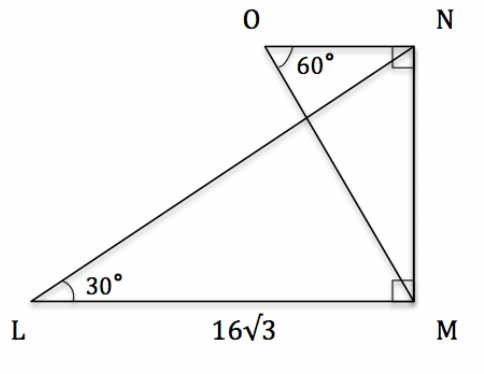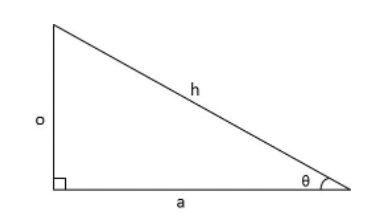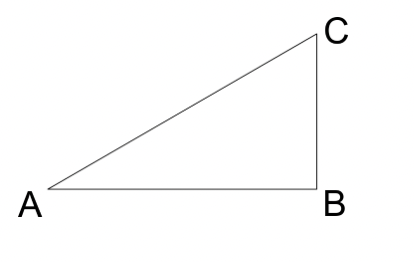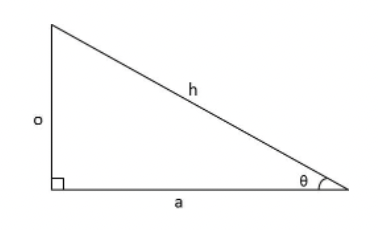# ACT Mathematics: Trignometery and Inequality Quiz

This is an MCQ-based quiz on the topic of ACT Mathematics: Trignometery and Inequality Quiz.

This set of MCQs helps you brush up on the important mathematics topic and prepare you to dive into skill practice and expand your knowledge to tackle the question and answer it carefully.

Start Quiz

For the triangles in the figure given, which of the following is closest to the length of line NO?7

6

9

10

In the above triangle, o=4 and a=7. Find θ.40

35

25

30

Triangle ABC shown is a right triangle. If the tangent of angle C is 37, what is the length of segment BC?6

7

8

9

What is the tangent line equation of

y=x^4+4x

at point

(1,5) ?

y=8x−3

y=8x+3

y=8x−8

y=8x+8

What is the tangent of C in the given right triangle??AB/BC

AB/AC

CB/AB

CB/AC

Give the equation, in slope-intercept form, of the line tangent to the circle of the equation

x^2+y^2=225

at the point (12,−9).

y = 4/3x−25

y = 4/3x+25

y = 4/3x−30

y = 4/3x-12

For the above triangle, o=14 and a=28. Find θ.27

30

35

20

If you multiply two integers together and then add 5, the result is 69. Which of the following could not be the sum of the two integers?

16

34

20

24

Quiz/Test Summary
Title: ACT Mathematics: Trignometery and Inequality Quiz
Questions: 8
Contributed by: